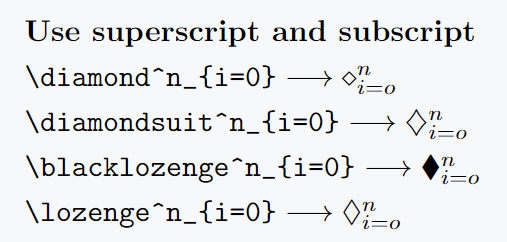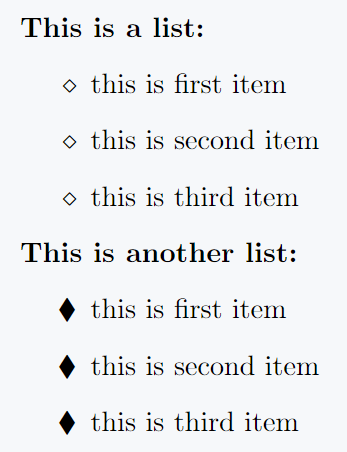# How do you represent Diamond(◇) symbol in LaTeX?

You can use the default command \diamond to print the diamond symbol in LaTeX. There are also more packages that provide you with different diamond symbols.

In this tutorial we will discuss how to use all kinds of diamond symbols in LaTeX.

Package command and output
None \diamond → ⋄
None \diamondsuit → ◇
amssymb \lozenge → ♢
amssymb \blacklozenge → ♦
stix \enclosediamond →   ⃟

## Diamond symbol with limits

Use the following syntax to use superscripts and subscripts with diamond products or diamond symbols.

\diamond^{superscript}_{subscript}

\documentclass{article}
\usepackage{amssymb}
\begin{document}
\textbf{Use superscript and subscript}\\[3pt]
$\verb|\diamond^n_{i=0}|\longrightarrow\diamond^n_{i=o}$\\[3pt]
$\verb|\diamondsuit^n_{i=0}|\longrightarrow\diamondsuit^n_{i=o}$\\[3pt]
$\verb|\blacklozenge^n_{i=0}|\longrightarrow\blacklozenge^n_{i=o}$\\[3pt]
$\verb|\lozenge^n_{i=0}|\longrightarrow\lozenge^n_{i=o}$
\end{document}

Output :## Use diamond symbol in bulleted lists

A typical bulleted listing uses the large dot symbol, but you can also use the diamond symbol if you prefer. Explained by example below

\documentclass{article}
\usepackage{amssymb,enumitem}
\begin{document}
\textbf{This is a list:}
\begin{itemize}[label=$\diamond$]
\item this is first item
\item this is second item
\item this is third item
\end{itemize}
\textbf{This is another list:}
\begin{itemize}[label=$\blacklozenge$]
\item this is first item
\item this is second item
\item this is third item
\end{itemize}
\end{document}

Output :#### Md Jidan Mondal

LaTeX expert with over 10 years of experience in document preparation and typesetting. Specializes in creating professional documents, reports, and presentations using LaTeX.